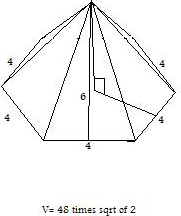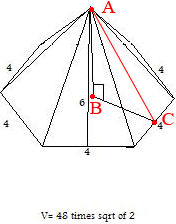SEARCH HOMEMath Central Quandaries & QueriesMy son is working on a hexagonal pyramid. The base is 4 and the slant height is 6. We know that the answer is 48 times the sqrt of 2. We don't know how they got it. We attached the drawing of it. Thanks AngieHi Angie,The volume of a pyramid is 1/3 × (the area of the base) × (the height) so you need to find the area of the base and the height.

You can find the area of the base using the technique Stephen used in his response to an earlier problem.

o find the height I added a line to your diagram as well as some labels.The height of the pyramid is the length of the side AB of the right triangle ABC. |AC| = 6 and |BC| you found in determining the area of the hexagonal base. Thus you can use Pythagoras theorem to find |AB|.

I hope this helps,
PennyMath Central is supported by the University of Regina and The Pacific Institute for the Mathematical Sciences.